## 3.8.1 Description and Postulates

 Suppose that we have entities distributed around the city in a completely random manner. These entities could be employees of a particular service system, recipients of a certain social service, emergency response units, crimes, and so on. We require a way of describing probabilistically the numbers of entities in given subareas and spatial interrelationships among entities. To do this, we generalize the idea of a Poisson process in time to a Poisson process in space. We recall from Chapter 2 that for a homogeneous Poisson process in time, the probability that exactly k Poisson events occur in a fixed time interval [0, t] is ```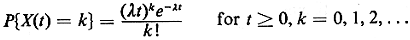``` where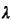is a positive constant interpreted as the average rate at which events are happening per unit time. The process is called "homogeneous," becausedoes not vary with time. Applying the same ideas in a spatial setting, first consider a homogeneous highway segment of length l miles. From past accident records we may know that each year an average ofhighway accidents occur per mile on this type of highway. Then the number of highway accidents that occur in the segment of length l miles can be modeled as a Poisson random variable with meanl, Here the parameter distance (l) plays a role directly analogous to time (t). For the Poisson model to be a reasonable one, the locations of accidents must occur consistent with Poisson- type assumptions: (1) only nonnegative integer numbers of accidents can occur in any length of highway; (2) the probability distribution of the number of accidents depends only on the length of highway considered, and as this length goes to zero so does the probability of an accident occurring there; (3) the numbers of accidents occurring in nonoverlapping segments of highway are mutually independent random variables; and (4) given that an accident occurs at a particular location, the chance of a second accident occurring at the identically same location is zero. Assumptions (1) and (3) appear fairly reasonable for most highways. Since different parts of a highway (e.g., curves versus straight-aways) can be associated with different risks of accident, the first part of assumption (2) may have to be modified in practice to allow for a spatially varying (nonhomogeneous) Poisson process, with accompanying(x) defined so that(x)dx = probability of an accident occurring (during a year) in the road interval x to x + dx. A highway with overpasses, bridges, and other discernible high-risk points may yield a positive probability of at least one accident during a year at these points (e.g., at the base of an overpass), thereby negating the second part of assumption (2). Such high-risk points would also tend to negate assumption (4), which also might be invalidated by chain-reaction multiple-car accidents (such as those that occasionally occur in fog) if those are counted in accident data as more than one accident. In practice, almost any real system will demonstrate a nonperfect degree of conformity with the postulates of the Poisson process. In assessing the applicability of the Poisson model, the modeler must weigh the benefits of applying the Poisson model (together with the insights it provides) against the cost of inaccuracies introduced by such a simple model and the cost of constructing a more complex model. Sometimes a forced degree of ignorance involving details of a system (e.g., the locations of overpasses) will facilitate application of the model. The ideas illustrated above for a Poisson process on a line (a highway) extend directly to the plane (to describe entities distributed over two dimensions). Let the parameter S denote a bounded region of the plane (or higher- dimensional space, for that matter). Let X(S) be the number of entities contained in S. Then X(S) is a homogeneous spatial Poisson process if it obeys the Poisson postulates, yielding a probability distribution `` In this caseis a positive constant called the intensity parameter of the process and A(S) represents the area or volume of S, depending on whether S is a region in the plane or higher-dimensional space. The underlying mathematical postulates of the model follow directly those of the time Poisson process: ```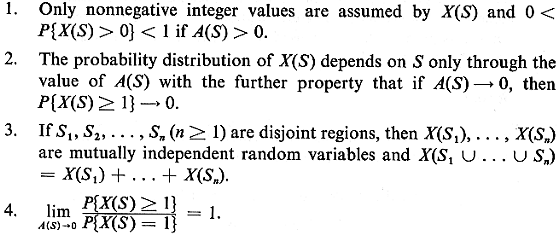``` Generalization: As with the time Poisson process, it is not difficult to extend these ideas to a spatially varying (nonhomogeneous) Poisson process. For instance, in the plane if(x, y)dxdy = P{a Poisson entity is located in the interval x to x + dx, y to y + dy} then in (3.96),A(S) is replaced by ```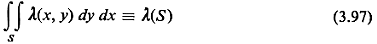``` In this case postulate (2) is changed to read: "The probability distribution of X(S) depends on S only through the value of(S) with the further property that as(S)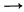0, then P{X(S)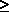1}0."